## Want to keep learning?

This content is taken from the Raspberry Pi Foundation & National Centre for Computing Education's online course, How Computers Work: Demystifying Computation. Join the course to learn more.
4.8

## Raspberry Pi Foundation

Skip to 0 minutes and 6 seconds This is the Little Man Computer, or LMC. It’s an instructional model of a computer created in around 1965. It models a simple Von Neumann architecture with all the basic features of a modern computer.

Skip to 0 minutes and 23 seconds We can program the LMC using machine code. Machine code is normally binary numbers that represent instructions to the computer. However, with the LMC, we use denary numbers. On the left hand side of the screen, you can see the Little Man Computer’s instruction set. The Xs are for addresses in RAM. So 550, for example, would be an instruction to load the number in address 50 into the accumulator. So let’s watch a little program using machine code. I’m going to start by placing two numbers into RAM. So in address 60 I’m going to put the number 5. And in address 61 I’ll put the number 3. The program is going to start at address 0.

Skip to 1 minute and 8 seconds I’m going to begin by loading the number in address 60. So that’s 560. Next, I want to add the number in address 61 to the number in the accumulator. So that’s 161. To finish off, I’m going to store the output of the calculation. And I’m going to put it in address 62. So that’s 362. Let’s run the program and see what happens. The program counter is currently at 0. So the instruction at address 0 is fetched and the program counter incremented. The instruction 560 now needs to be decoded. The computer now knows to fetch the value at address 60. This is placed in the accumulator. It now fetches the next instruction. And the program counter is incremented again.

Skip to 1 minute and 54 seconds So the instruction 161 needs to be decoded. That means fetching the number at 61, placing it into the accumulator, and the ALU then adding them together to give an 8. The instruction address two is now fetched, which is 362. This is decoded. And the number from the accumulator is taken and stored in address 62. Finally, it goes to the instruction at address three, which is 000. This is machine code for halt. So my program stops. But it has successfully added two numbers and stored the result. Even though we only have 10 instructions in the instruction set, we can still create quite complicated programs using the Little Man Computer. Here is a program with 16 instructions used in total.

Skip to 2 minutes and 45 seconds The first instruction, 901, fetches some user input. And I’m going to input the number 5. Now, this program will take about 10 minutes to run normally. So I’m going to speed it up. So we can see the final output. You’ll notice that as the program runs, the program counter sometimes goes back to an earlier number. This is an indication of branching. The program is moving forwards and then going backwards and repeating some of the earlier instructions. What it’s actually doing is taking the number that’s input and adding it to itself a certain number of times. If we look at the final output of this program, we can see that it is the number 25. So this program squares user input.

Skip to 3 minutes and 32 seconds In the next few steps, we’ll take a closer look at programming with machine code and give you some challenges to program yourself.

# The Little Man Computer

This is the ‘little man computer’, or LMC. It’s an instructional model of a computer, created in around 1965.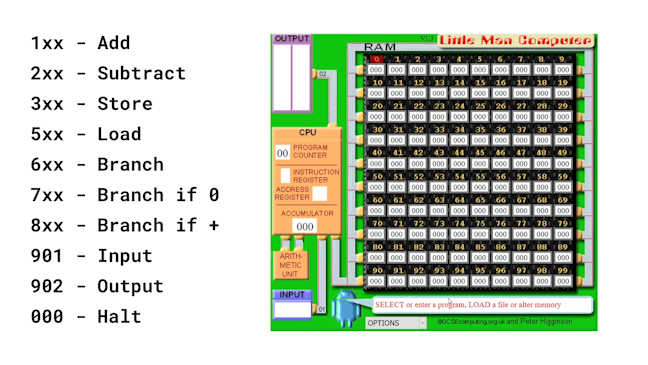It models a simple Von Neumann architecture, with all the basic features of a modern computer. We can program the LMC using ‘machine code’. Machine code is normally binary numbers that represent instructions to the computer. However, with the LMC, we use denary numbers.

On the left hand side of the image, you can see the Little Man Computer’s instruction set. The x’s are for addresses in RAM. For example, 5xx means load, so 550 would be the instruction to load the number in address 50 into the accumulator.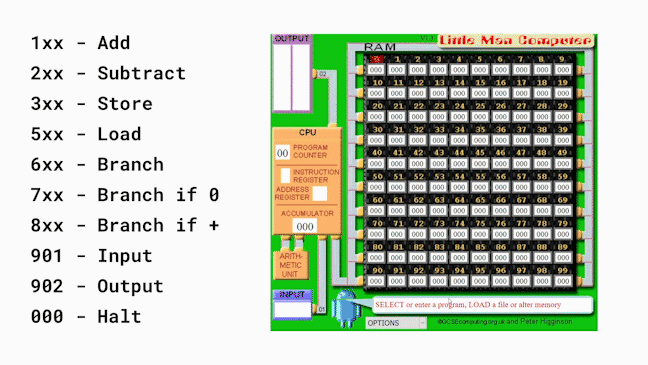Here’s how we could write a little program using machine code. We’ll start by placing two numbers into RAM. In address 60, we’ll put the number 5, and in address 61, the number 3. The program itself is going to start at address 0.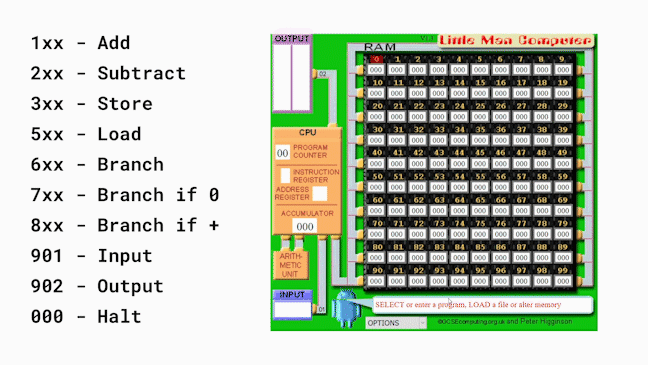We want the program to begin by loading the number in address 60. So, the first instruction must be 560, which we put into address 0.

Next, we want to add the number in address 61 to the number in the accumulator. So, the second instruction is 161, which we write in address 1.

To finish off, we store the output of the calculation; let’s put it in address 62. So the instruction 362 stored in address 2.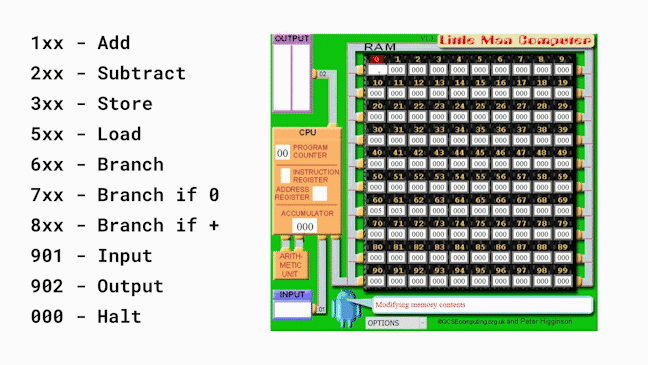Here’s a close up of the finished program and data.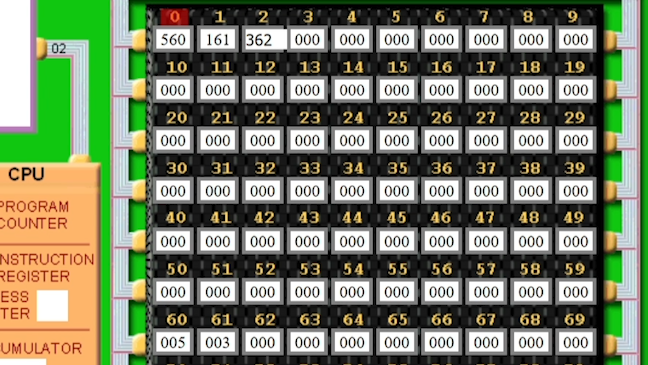Let’s run the program and see what happens…

• The program counter is currently at 0. So the instruction at address 0 is fetched and the program counter incremented.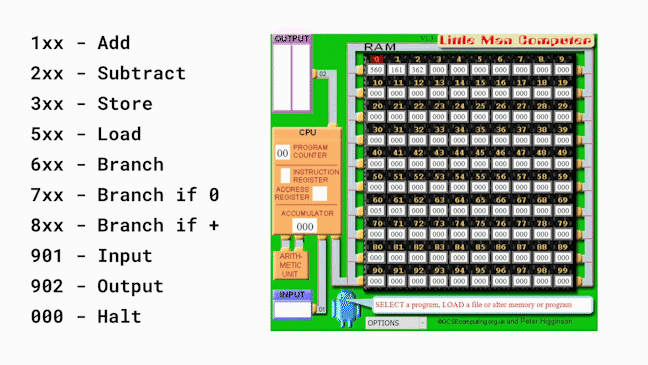• By decoding the instruction 560, the computer now knows to fetch the value at address 60. This is placed in the accumulator.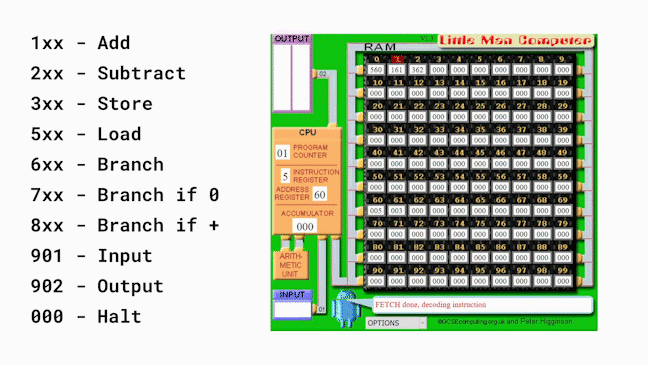• It now fetches the next instruction and the program counter is incremented again, so the instruction 161 needs to be decoded.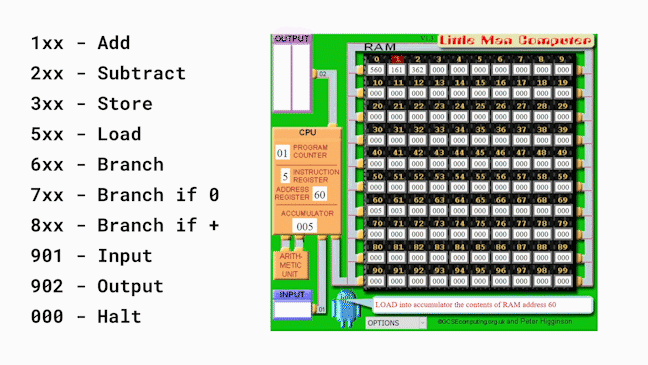• That means fetching the number at 61, placing it into the accumulator, and then sending both numbers to the ALU which adds them together. The result is 8, which is stored in the accumulator.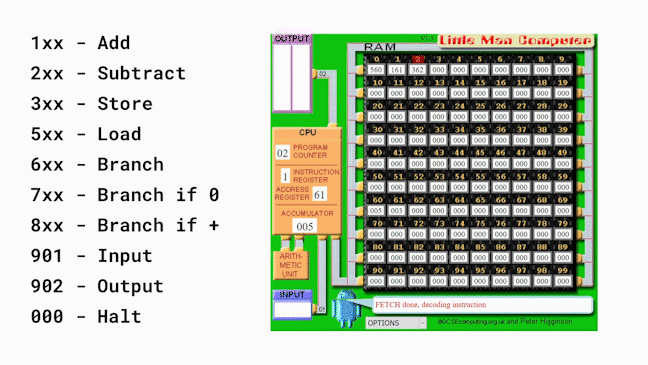• The instruction at address 2 is now fetched, which is 362.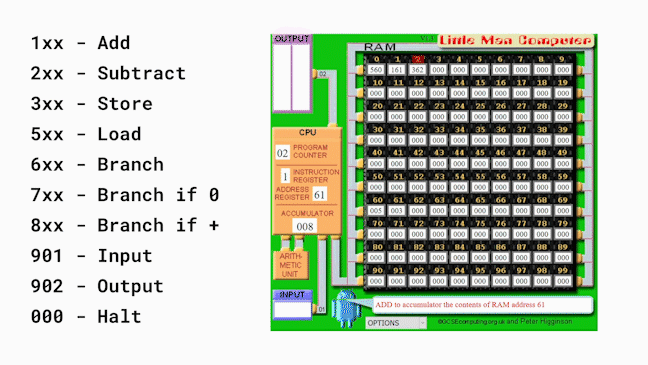• This is decoded as a store instruction, so the number from the accumulator is taken and stored in address 62.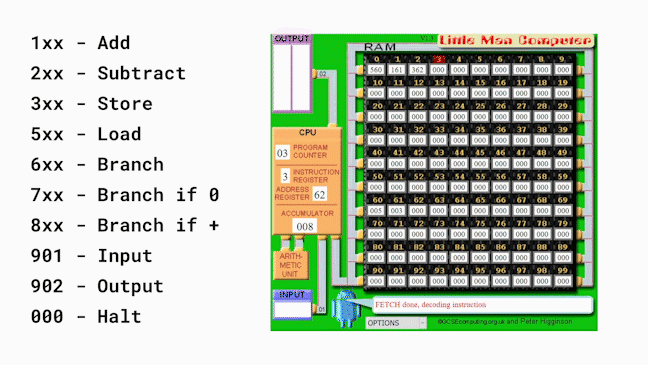• Finally, the computer goes to the instruction at address 3, which is 000. This is the machine code for ‘halt’, so the program stops. It has successfully added two numbers and stored the result.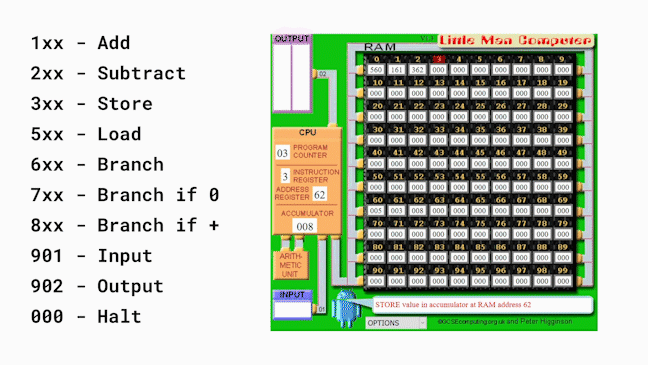Even though the Little Man Computer only has ten instructions in its instruction set, we can still create quite complicated programs for it.

Here is a program with sixteen instructions used in total.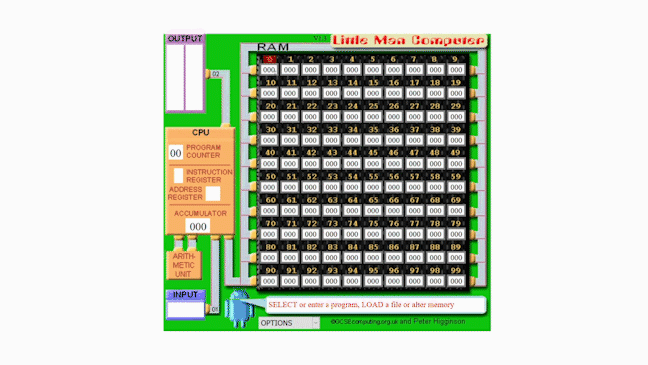The first instruction 9-01, fetches some input from the user. I’m going to input the number 5.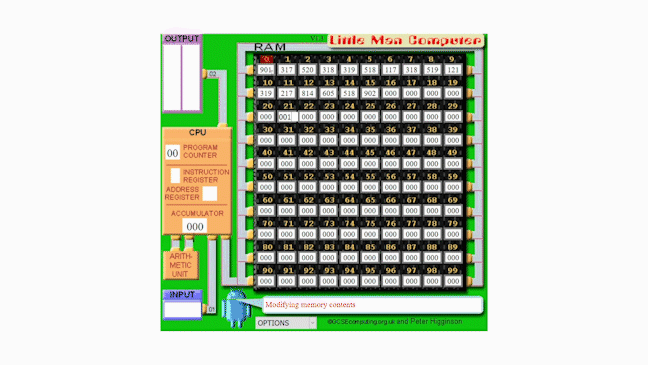This program would take about 10 minutes to run at the speed of the simulations above, so instead, here is a speeded-up version so that you can see the final output.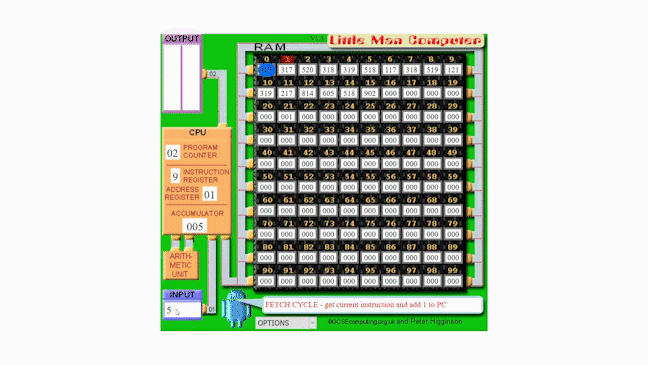You’ll notice that as the program runs, the program counter sometimes goes back to an earlier number. This is an example of branching. Sometimes an instruction changes the program counter, so the program goes back to an earlier instruction. This enables a sequence of instructions to be repeated a number of times.

If we look at the final output of this program, we can see that the number is 25. In fact, this program squares the number that was input by the user. As I input 5, the final output is 5x5 = 25.

In the next few steps, we’ll take a closer look at programming the little man computer and give you some challenges to program yourself.